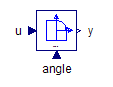DCV 43 Generic - MapleSim Help

DCV 43 Generic

4-way 3-position valve with user-specified flow pathsDescription The DCV 43 Generic component is a four-port, three-position valve that allows flow between the ports based on the user-specified custom flow path. A generic form allows the user to specify the custom scenario according to the need.  The user can choose between either of the two options specified below. 1. Characteristic Curve - By choosing this option, user-supplied characteristic data which defines the relationship between the spool signal and the spool opening is specified. A suboption under this category is that the spool opening can either be given as area or as a scaled opening by toggling between Use scales options. 2. Flow path - Flow paths with respect to the spool signal where the input s controls the position of the value. An input of 0 corresponds to position 0, an input of 1 to position 1. There are three orifices inter-connected to each other which open up according to the input feed thereby allowing fluid to flow between the different port. Intermediates values (between 0 and 1) produce an averaged position, with the transition length set by the $\mathrm{band}$ parameter. Based on the orifice area, the pressure vs. flow rate relationship is calculated by the formulation used in the Orifice component.Spool Signal vs Valve Opening For the characteristic curve option component, the area of opening or scaled coefficient must be specified as a function of the $\mathrm{Spool}$ signal. The four columns in the data correspond to order (Signal - Spool PA - Spool TA - Spool PT) specified in the form of a table. The following parameters allows users to specify the necessary data for this calculation. Data Source: This parameter allows users to specify the nature of the source file for the Signal (ranging from -1 to 1) vs. valve opening table. The available options are file, attachment, and inline. file: Use this option to specify a source file (an Excel or a CSV file) located on the hard drive. Use the S(opening); attachment: Use this option to specify a data file (an Excel or a CSV file) that is attached to the MapleSim model. To be used as an attachment, data files must be attached to the MapleSim model and be categorized under Data Sets. The attached files in a MapleSim model can be viewed in the Attachments pane under the Data Sets section. For more information, see Attaching a File to a Model. If the required file is not already attached to the MapleSim model, use the $S\left(\mathrm{opening}\right)$, or if the use scales option is unchecked then $S\left(A\right)$ parameter to attach a new file. Note: If the file option is used, subsequent changes to the source file will be incorporated into MapleSim simulations. If the attachment option is used, subsequent changes to the source file will not affect the version attached to the MapleSim model. To ensure updates to the source files are incorporated in the simulation, the file must be reattached. inline: Use this option to directly specify the pressure vs. area relationship in the form of a table. In the $S\left(\mathrm{opening}\right)$or $S\left(\mathrm{Area}\right)$ parameter the first column must contain values of the spool signal ranging from -1 to 1 and the second column must contain corresponding values of the area or scaled area. Note: To change the dimensions of table, right-click (Control-click for Mac®) the parameter field and select Edit Matrix Dimensions. In the Matrix Dimensions dialog, enter values for the number or rows and columns and then click OK. Smooth: The smooth option specifies how the data table is interpolated.Connections

 Name Description Modelica ID $\mathrm{portA}$ Hydraulic port A portA $\mathrm{portB}$ Hydraulic port B portB $\mathrm{portP}$ Hydraulic port P (pump) portP $\mathrm{portT}$ Hydraulic port T (tank) portT $s$ Spool input signal sParametersGeneral

X and Y correspond to ports A, B, P, and T.

 Name Default Units Description Modelica ID Characteristic curves $\mathrm{false}$ True (checked) enables the Data parameters CharacteristicCurves use scales $\mathrm{true}$ True (checked) means the orifice maximum areas are the product of ${A}_{\mathrm{max}}$ and the scaling coefficients useScales ${A}_{\mathrm{max}}$ $1·{10}^{-4}$ ${m}^{2}$ Maximum orifice opening area; available when use scales is true. Amax ${A}_{\mathrm{min}}$ $1·{10}^{-12}$ ${m}^{2}$ Minimum orifice opening area (leakage) Amin ${s}_{\mathrm{X-Y}}$ Scaling coefficients, see  sXY ${\mathrm{area}}_{\mathrm{X-Y}}$ ${m}^{2}$ Areas, see  aXY $\mathrm{band}$ $0.1$ Transition length in normalized spool displacement ranges from 0.1 - 0.9; see  band

 ${s}_{\mathrm{X-Y}}$ is a two-element vector that specifies the scaling coefficient between ports X and Y when the $s$ input is 0 and 1, respectively. The opening area is computed from ${A}_{\mathrm{min}}$, ${A}_{\mathrm{max}}$, $s$, and ${s}_{\mathrm{X-Y}}$. Available when Characteristic curves is false and use scales is true.

 ${\mathrm{area}}_{\mathrm{X-Y}}$ is a two-element vector that specifies the area between ports X and Y when the $s$ input is 0 and 1, respectively. Available when Characteristic curves and use scales are false.

  Available when Characteristic curves is false.Data

These parameters are enabled when Characteristic curves is true. They use a matrix to interpolate the area or scaling coefficients from the spool input signal, $s$, depending on whether

 Name Default Units Description Modelica ID data source $\mathrm{inline}$ Selects how the matrix is assigned datasourcemode $S$ Defines ${s}_{\mathrm{X-Y}}$ vs $s$. Columns: $s,{s}_{\mathrm{P-A}},{s}_{\mathrm{T-A}},{s}_{\mathrm{P-T}}$. Available if use scales is true. S $A$ ${m}^{2}$ Defines ${\mathrm{area}}_{\mathrm{X-Y}}$ vs $s$. Columns: $s,{\mathrm{area}}_{\mathrm{P-A}},{\mathrm{area}}_{\mathrm{T-A}},{\mathrm{area}}_{\mathrm{P-T}}$. Available if use scales is false. A $\mathrm{Smoothness}$ $\mathrm{linear}$ Smoothness of table interpolation SmoothnessOrifice

 Name Default Units Description Modelica ID $\mathrm{Use constant Cd}$ $\mathrm{true}$ True (checked) means a constant coefficient of discharge is implemented, otherwise a variable ${C}_{d}$ is used in flow calculation UseConstantCd ${C}_{d}$ $0.7$ Flow-discharge coefficient; used when $\mathrm{Use constant Cd}$ is true Cd ${\mathrm{\Re }}_{\mathrm{Cr}}$ $12$ Reynolds number at critical flow; used when $\mathrm{Use constant Cd}$ is true ReCr ${C}_{d\left(\mathrm{max}\right)}$ $0.7$ Maximum flow-discharge coefficient; used when $\mathrm{Use constant Cd}$ is false Cd_max ${\mathrm{Crit}}_{\mathrm{no}}$ $1000$ Critical flow number; used when $\mathrm{Use constant Cd}$ is false Crit_noOptional Volumes

X corresponds to ports A, B, P, and T.

 Name Default Units Description Modelica ID $\mathrm{Use volume X}$ $\mathrm{false}$ True (checked) means a hydraulic volume chamber is added to portX useVolumeX ${V}_{X}$ $1·{10}^{-6}$ ${m}^{3}$ Volume of chamber attached to portX Vx